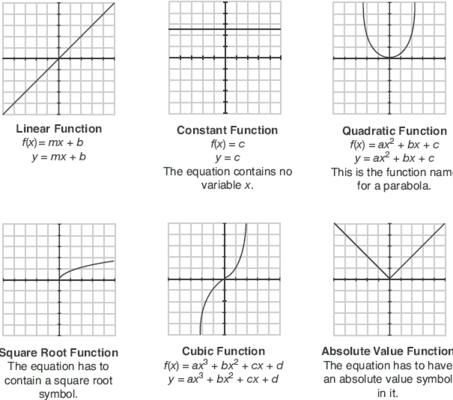Parent Functions
3 years ago
teamerdmann
Save
Edit
Host a game
Live GameLive
Homework
Solo Practice
Practice20 QuestionsShow answers
• Question 1
180 secondsQ. Which parent function matches the graph?
y = |x|
y = ∛(x)
y = x2
y = a⋅bx
• Question 2
60 secondsQ. What type of function is shown?
absolute value
linear
exponential
• Question 3
60 secondsQ. What type of function is shown?
linear
square root
absolute value
• Question 4
60 secondsQ. Which parent function matches the graph?
f(x) = x
f(x)= x2
f(x) = |x|
f(x) = abx
• Question 5
60 secondsQ. Which parent function matches the graph?
f(x) = x
f(x)= x2
f(x) = |x|
f(x) = abx
• Question 6
60 secondsQ. What type of function is shown?
exponential
linear
square root
• Question 7
60 secondsQ. What type of function is shown?
Exponential
square root
cube root
• Question 8
60 secondsQ. What type of function is shown?
exponential
linear
square root
• Question 9
60 secondsQ. Which parent function matches the graph?
f(x) = x
f(x) = x2
f(x) =√(x)
f(x) =∛(x)
• Question 10
60 secondsQ. Which parent function matches the graph?
f(x) = a⋅bx
f(x) = ∛(x)
f(x) = √(x)
f(x) = x2
• Question 11
60 secondsQ. Which parent function matches the graph?
f(x) = √(x)
f(x) = ∛(x)
f(x) = |x|
f(x) = a⋅bx
• Question 12
180 secondsQ. Name the parent function.
constant
linear
exponential
• Question 13
180 secondsQ. Name the parent function.
constant
square root
linear
absolute value
• Question 14
30 seconds
Q. g(x)=f(x)-4
Describe the transformation to f(x) that results in g(x).
f(x) has been translated down 4.
f(x) has been translated up 4.
• Question 15
30 seconds
Q. g(x)=f(x)+6
Describe the transformation to f(x) that results in g(x).
f(x) has been translated up 6.
f(x) has been translated down 6.
• Question 16
30 seconds
Q. g(x)=f(x-4)
Describe the transformation to f(x) that results in g(x).
f(x) has been translated to the right 4.
f(x) has been translated to the left 4.
• Question 17
30 seconds
Q. g(x)=f(x-3)
Describe the transformation to f(x) that results in g(x).
f(x) has been translated to the right 3.
f(x) has been translated to the left 3.
• Question 18
30 seconds
Q. g(x)=f(x+5)
Describe the transformation to f(x) that results in g(x).
f(x) has been translated to the left 5.
f(x) has been translated to the right 5.
• Question 19
30 seconds
Q. How did the equation shift from the parent function? y=x+2
up 2
down 2
steeper
flipped
• Question 20
30 secondsQ. Match the equation to its description.